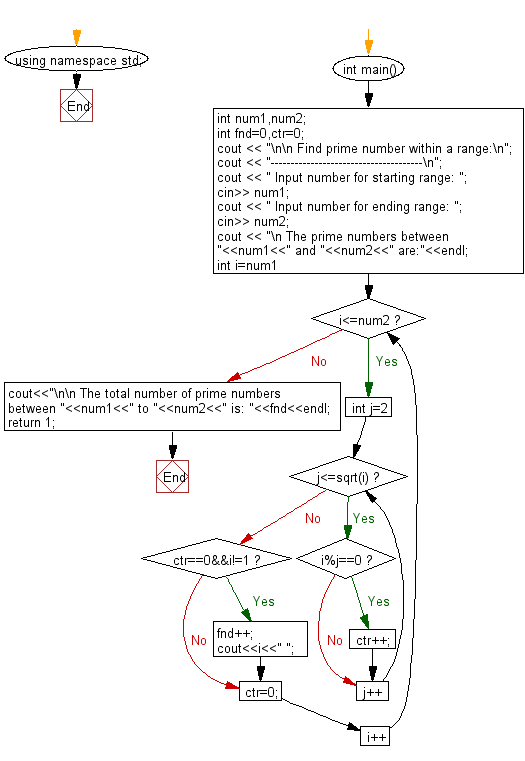﻿ C++ Exercises: Find prime number within a range - w3resource

# C++ Exercises: Find prime number within a range

## C++ For Loop: Exercise-6 with Solution

Write a program in C++ to find a prime number within a range.

Sample Solution:

C++ Code:

``````#include <iostream>
#include<math.h>
using namespace std;
int main()
{
int num1,num2;
int fnd=0,ctr=0;
cout << "\n\n Find prime number within a range:\n";
cout << "--------------------------------------\n";
cout << " Input number for starting range: ";
cin>> num1;
cout << " Input number for ending range: ";
cin>> num2;

cout << "\n The prime numbers between "<<num1<<" and "<<num2<<" are:"<<endl;
for(int i=num1;i<=num2;i++)
{
for(int j=2;j<=sqrt(i);j++)
{
if(i%j==0)
ctr++;
}
if(ctr==0&&i!=1)
{ fnd++;
cout<<i<<" ";

}
ctr=0;
}
cout<<"\n\n The total number of prime numbers between "<<num1<<" to "<<num2<<" is: "<<fnd<<endl;
return 1;
}
``````

Sample Output:

``` Find prime number within a range:
--------------------------------------
Input number for starting range: 1
Input number for ending range: 100

The prime numbers between 1 and 100 are:
2 3 5 7 11 13 17 19 23 29 31 37 41 43 47 53 59 61 67 71 73 79 83 89 97

The total number of prime numbers between 1 to 100 is: 25
```

Flowchart:C++ Code Editor: